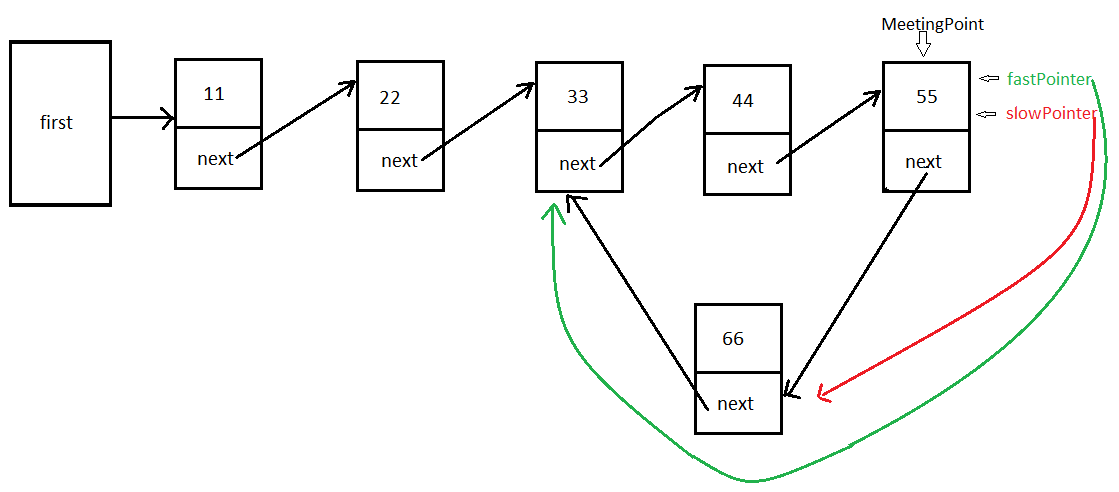### Single circular LinkedList - Calculate number of nodes in circular LinkedList in java

In this Data structures tutorial we are going to find/calculate number of nodes in circular Singly  LinkedList in java.

>After calculating number of nodes from  first to intersection point, we got to calculate number of nodes in loop.

Important methods used to find number of nodes in circular Singly  LinkedList in java are as follows>
>calculateNodesInCircularLikedList()

Logic explanation to find number of nodes in circular Singly  LinkedList in java with diagram >

After detection of meeting point make:
>slowPointer step over LinkedList by 1 Node
>fastPointer step over LinkedList by 2 Node

Repeat until both the pointers meet together. Keep a count of number of number nodes iterated which will give us length of the loop.Example to find number of nodes in circular Singly  LinkedList in java(considering above diagram)>

NOW WE HAVE TO FIND  MEETING POINT:
 slowPointer (steps 1node) fastPointer (steps 2node) start at 11 start at 11 >reaches 22 reaches 33 >reaches 33 reaches 55 >reaches 44 reaches 33 reaches 55 (meeting point) reaches 55 (meeting point)

NOW, LETS CALCULATE NUMBER OF ELEMENTS IN LOOP:
 slowPointer (steps 1node) fastPointer (now steps 2node) Iterations keep it on meeting point ( i.e.55) keep it on meeting point ( i.e.55) - >reaches 66 reaches 33 +1 >reaches 33 reaches 55 +1 >reaches 44 reaches 33 +1 >reaches 55(again both pointers have reached meeting point) reaches 55(again both pointers have reached meeting point) +1 Total=4
Therefore, number of nodes in loop are =4.

NOW, LETS CALCULATE NUMBER OF ELEMENTS FROM FIRST TO INTERSECTION POINT:
 slowPointer (steps 1node) fastPointer (now steps 1node) Iterations make it point to first (i.e.11) keep it on meeting point ( i.e.55) - >reaches 22 reaches 66 +1 >reaches 33 (intersection point) reaches 33 (intersection point) +1 Total=2
Therefore, number of nodes from first to intersection point are=2

Hence, if we sum up >
(number of nodes in loop) + (number of nodes from first to intersection point) = 4 + 2 = 6

Full Program/SourceCode/ Example to find number of nodes in circular Singly  LinkedList in java >

So in this Data structures tutorial we learned how to calculate number of nodes in circular Singly  LinkedList in java. This is also a very important java interview question.

Having any doubt? or you you liked the tutorial! Please comment in below section.

1) Stacks, Queues in Data Structures in java

2) Single LinkedList implementations in Data Structures in java:-

3) Doubly LinkedList implementations in Data Structures in java:-

5) Some of the tricky and interesting Single LinkedList implementations in Data Structures in java

6) Binary tree tutorial in java >